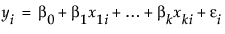Publication date: 07/30/2020

# The Factor Models

The way the x-variables (factors) are modeled to predict an expected value or probability is the subject of the factor side of the model.

The factors enter the prediction equation as a linear combination of x values and the parameters to be estimated. For a continuous response model, where i indexes the observations and j indexes the parameters, the assumed model for a typical observation, yi, is writtenwhere

yi is the response

xij are functions of the data

εi is an unobservable realization of the random error

bj are unknown parameters to be estimated.

The way the x’s in the linear model are formed from the factor terms is different for each modeling type. The linear model x’s can also be complex effects such as interactions or nested effects. Complex effects are discussed in detail later.